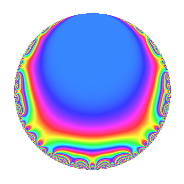# Properties

 Label 51.2.d.bLevel 51 Weight 2 Character orbit 51.d Analytic conductor 0.407 Analytic rank 0 Dimension 2 CM No Inner twists 2

# Related objects

## Newspace parameters

 Level: $$N$$ = $$51 = 3 \cdot 17$$ Weight: $$k$$ = $$2$$ Character orbit: $$[\chi]$$ = 51.d (of order $$2$$ and degree $$1$$)

## Newform invariants

 Self dual: No Analytic conductor: $$0.407237050309$$ Analytic rank: $$0$$ Dimension: $$2$$ Coefficient field: $$\Q(\sqrt{-1})$$ Coefficient ring: $$\Z[a_1, a_2, a_3]$$ Coefficient ring index: $$1$$ Sato-Tate group: $\mathrm{SU}(2)[C_{2}]$

## $q$-expansion

Coefficients of the $$q$$-expansion are expressed in terms of $$i = \sqrt{-1}$$. We also show the integral $$q$$-expansion of the trace form.

 $$f(q)$$ $$=$$ $$q$$ $$+ q^{2}$$ $$+ i q^{3}$$ $$- q^{4}$$ $$+ i q^{6}$$ $$-4 i q^{7}$$ $$-3 q^{8}$$ $$- q^{9}$$ $$+O(q^{10})$$ $$q$$ $$+ q^{2}$$ $$+ i q^{3}$$ $$- q^{4}$$ $$+ i q^{6}$$ $$-4 i q^{7}$$ $$-3 q^{8}$$ $$- q^{9}$$ $$+ 4 i q^{11}$$ $$-i q^{12}$$ $$+ 2 q^{13}$$ $$-4 i q^{14}$$ $$- q^{16}$$ $$+ ( 1 + 4 i ) q^{17}$$ $$- q^{18}$$ $$-4 q^{19}$$ $$+ 4 q^{21}$$ $$+ 4 i q^{22}$$ $$-4 i q^{23}$$ $$-3 i q^{24}$$ $$+ 5 q^{25}$$ $$+ 2 q^{26}$$ $$-i q^{27}$$ $$+ 4 i q^{28}$$ $$-4 i q^{31}$$ $$+ 5 q^{32}$$ $$-4 q^{33}$$ $$+ ( 1 + 4 i ) q^{34}$$ $$+ q^{36}$$ $$+ 8 i q^{37}$$ $$-4 q^{38}$$ $$+ 2 i q^{39}$$ $$-8 i q^{41}$$ $$+ 4 q^{42}$$ $$-4 q^{43}$$ $$-4 i q^{44}$$ $$-4 i q^{46}$$ $$-8 q^{47}$$ $$-i q^{48}$$ $$-9 q^{49}$$ $$+ 5 q^{50}$$ $$+ ( -4 + i ) q^{51}$$ $$-2 q^{52}$$ $$+ 6 q^{53}$$ $$-i q^{54}$$ $$+ 12 i q^{56}$$ $$-4 i q^{57}$$ $$-12 q^{59}$$ $$-8 i q^{61}$$ $$-4 i q^{62}$$ $$+ 4 i q^{63}$$ $$+ 7 q^{64}$$ $$-4 q^{66}$$ $$+ 12 q^{67}$$ $$+ ( -1 - 4 i ) q^{68}$$ $$+ 4 q^{69}$$ $$+ 12 i q^{71}$$ $$+ 3 q^{72}$$ $$+ 8 i q^{74}$$ $$+ 5 i q^{75}$$ $$+ 4 q^{76}$$ $$+ 16 q^{77}$$ $$+ 2 i q^{78}$$ $$-4 i q^{79}$$ $$+ q^{81}$$ $$-8 i q^{82}$$ $$+ 12 q^{83}$$ $$-4 q^{84}$$ $$-4 q^{86}$$ $$-12 i q^{88}$$ $$-10 q^{89}$$ $$-8 i q^{91}$$ $$+ 4 i q^{92}$$ $$+ 4 q^{93}$$ $$-8 q^{94}$$ $$+ 5 i q^{96}$$ $$+ 16 i q^{97}$$ $$-9 q^{98}$$ $$-4 i q^{99}$$ $$+O(q^{100})$$ $$\operatorname{Tr}(f)(q)$$ $$=$$ $$2q$$ $$\mathstrut +\mathstrut 2q^{2}$$ $$\mathstrut -\mathstrut 2q^{4}$$ $$\mathstrut -\mathstrut 6q^{8}$$ $$\mathstrut -\mathstrut 2q^{9}$$ $$\mathstrut +\mathstrut O(q^{10})$$ $$2q$$ $$\mathstrut +\mathstrut 2q^{2}$$ $$\mathstrut -\mathstrut 2q^{4}$$ $$\mathstrut -\mathstrut 6q^{8}$$ $$\mathstrut -\mathstrut 2q^{9}$$ $$\mathstrut +\mathstrut 4q^{13}$$ $$\mathstrut -\mathstrut 2q^{16}$$ $$\mathstrut +\mathstrut 2q^{17}$$ $$\mathstrut -\mathstrut 2q^{18}$$ $$\mathstrut -\mathstrut 8q^{19}$$ $$\mathstrut +\mathstrut 8q^{21}$$ $$\mathstrut +\mathstrut 10q^{25}$$ $$\mathstrut +\mathstrut 4q^{26}$$ $$\mathstrut +\mathstrut 10q^{32}$$ $$\mathstrut -\mathstrut 8q^{33}$$ $$\mathstrut +\mathstrut 2q^{34}$$ $$\mathstrut +\mathstrut 2q^{36}$$ $$\mathstrut -\mathstrut 8q^{38}$$ $$\mathstrut +\mathstrut 8q^{42}$$ $$\mathstrut -\mathstrut 8q^{43}$$ $$\mathstrut -\mathstrut 16q^{47}$$ $$\mathstrut -\mathstrut 18q^{49}$$ $$\mathstrut +\mathstrut 10q^{50}$$ $$\mathstrut -\mathstrut 8q^{51}$$ $$\mathstrut -\mathstrut 4q^{52}$$ $$\mathstrut +\mathstrut 12q^{53}$$ $$\mathstrut -\mathstrut 24q^{59}$$ $$\mathstrut +\mathstrut 14q^{64}$$ $$\mathstrut -\mathstrut 8q^{66}$$ $$\mathstrut +\mathstrut 24q^{67}$$ $$\mathstrut -\mathstrut 2q^{68}$$ $$\mathstrut +\mathstrut 8q^{69}$$ $$\mathstrut +\mathstrut 6q^{72}$$ $$\mathstrut +\mathstrut 8q^{76}$$ $$\mathstrut +\mathstrut 32q^{77}$$ $$\mathstrut +\mathstrut 2q^{81}$$ $$\mathstrut +\mathstrut 24q^{83}$$ $$\mathstrut -\mathstrut 8q^{84}$$ $$\mathstrut -\mathstrut 8q^{86}$$ $$\mathstrut -\mathstrut 20q^{89}$$ $$\mathstrut +\mathstrut 8q^{93}$$ $$\mathstrut -\mathstrut 16q^{94}$$ $$\mathstrut -\mathstrut 18q^{98}$$ $$\mathstrut +\mathstrut O(q^{100})$$

## Character Values

We give the values of $$\chi$$ on generators for $$\left(\mathbb{Z}/51\mathbb{Z}\right)^\times$$.

 $$n$$ $$35$$ $$37$$ $$\chi(n)$$ $$1$$ $$-1$$

## Embeddings

For each embedding $$\iota_m$$ of the coefficient field, the values $$\iota_m(a_n)$$ are shown below.

For more information on an embedded modular form you can click on its label.

Label $$\iota_m(\nu)$$ $$a_{2}$$ $$a_{3}$$ $$a_{4}$$ $$a_{5}$$ $$a_{6}$$ $$a_{7}$$ $$a_{8}$$ $$a_{9}$$ $$a_{10}$$
16.1
 − 1.00000i 1.00000i
1.00000 1.00000i −1.00000 0 1.00000i 4.00000i −3.00000 −1.00000 0
16.2 1.00000 1.00000i −1.00000 0 1.00000i 4.00000i −3.00000 −1.00000 0
 $$n$$: e.g. 2-40 or 990-1000 Significant digits: Format: Complex embeddings Normalized embeddings Satake parameters Satake angles

## Inner twists

Char. orbit Parity Mult. Self Twist Proved
1.a Even 1 trivial yes
17.b Even 1 yes

## Hecke kernels

This newform can be constructed as the kernel of the linear operator $$T_{2}$$ $$\mathstrut -\mathstrut 1$$ acting on $$S_{2}^{\mathrm{new}}(51, [\chi])$$.